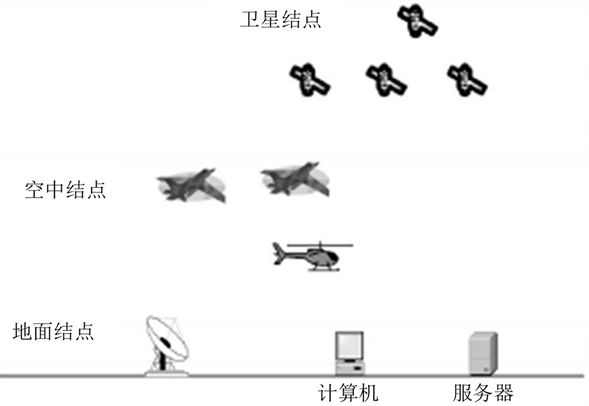# 空间网络中密钥协商协议的设计与分析The Design and Analysis of Key Agreement Protocol in Space Information Network

DOI: 10.12677/CSA.2018.86114, PDF, HTML, XML, 下载: 553  浏览: 2,118

Abstract: In order to meet the security and efficient communication requirements of nodes in space information network, a scheme of key agreement between nodes based on combined public key is proposed. The ground control center first completes the generation of the combination key based on identity and distributes it to the space nodes through a secure channel. When communication between different nodes is needed, after the two-way authentication is carried out, the two party nodes' session key is calculated by using their three secret values and the trusted public key information of the other nodes. The security properties of the protocol are analyzed, and compare the protocol and related protocols existing in the two aspects of security and performance. The comparison results show that this protocol not only has improved in terms of safety, and has higher computational efficiency.

1. 引言

2. 认证密钥协商协议

2.1. 系统结构Figure 1. The topology of the space network system

2.2. 系统初始化

1) 生成一个k比特的素数p，选择阶为q的有限域 ${F}_{p}$ 。在 ${F}_{p}$ 上选择两个数 $a,b\in {F}_{p}$ ，满足 $4{a}^{3}+27{b}^{2}\ne 0\mathrm{mod}\text{}p$ ，以 $a,b$ 为参数，生成椭圆曲线： $E\left(a,b\right):{y}^{2}\equiv {x}^{3}+ax+b\mathrm{mod}p$

2) 在 $E\left(a,b\right)$ 上选择一个阶为素数n的基点P，根据相应算法随机选取 ${x}_{ij}\in GF\left(p\right)$ ，其中 $1\le i\le m,1\le j\le n,m\in {Z}^{+},n\in {Z}^{+}$ 。构造 $m×n$ 的私钥种子矩阵 ${X}_{PR}$ ,有

${X}_{PR}=\left[\begin{array}{cccc}{x}_{11}& {x}_{12}& \cdots & {x}_{1n}\\ {x}_{21}& {x}_{22}& \cdots & {x}_{2n}\\ ⋮& ⋮& & ⋮\\ {x}_{m1}& {x}_{m2}& \cdots & {x}_{mn}\end{array}\right]$ (1)

${Y}_{PR}=\left[\begin{array}{cccc}{y}_{11}& {y}_{12}& \cdots & {y}_{1n}\\ {y}_{21}& {y}_{22}& \cdots & {y}_{2n}\\ ⋮& ⋮& & ⋮\\ {y}_{m1}& {y}_{m2}& \cdots & {y}_{mn}\end{array}\right]$ (2)

3) 选择两个单向哈希数 ${H}_{1}:{\left\{0,1\right\}}^{*}\to {Z}_{n}^{*};{H}_{2}:{\left\{0,1\right\}}^{*}\to {Z}_{p}^{*}$ ，完成系统初始化，向所有节点成员公开参数 $\left\{E\left(a,b\right),{X}_{PR},{Y}_{Pk},P,{H}_{1},{H}_{2}\right\}$

2.3 密钥种子对的生成

1) 根据公式 ${H}_{1}\left(ID\right)={h}_{1}\cdots {h}_{i}\cdots {h}_{n}$ ，计算出节点的散列值， ${h}_{i}$ 是长度为1的二进制比特串，转化成十进制数为 ${a}_{i}$ ，容易知道 $i\in \left[l,n\right],{a}_{i}\in \left[0,m\right)$

2) 从2.2中的 ${X}_{PR}$ 矩阵中选出节点私钥种子 $Seed{X}_{ID}=\left\{{x}_{{a}_{l},l},\cdots ,{x}_{{a}_{i}i},\cdots ,{x}_{{a}_{n}n}\right\}$ ，其中 ${x}_{{a}_{i}i}$ 为私钥种子矩阵 ${X}_{PR}$ 中第 ${a}_{i}$ 行，第i列对应的值。

3) 从2.2节中的 ${Y}_{PR}$ 中选取公钥种子 $Seed{Y}_{ID}=\left\{{y}_{{a}_{1},1},\cdots ,{y}_{{a}_{i}i},\cdots ,{y}_{{a}_{n}n}\right\}$ ，其中 ${y}_{{a}_{i}i}$${Y}_{PR}$ 中第 ${a}_{j}$ 行，第j列对应的值。

4) 将节点的公私钥种子对 $\left(Seed{X}_{ID},Seed{Y}_{ID}\right)$ 存储到对应节点，同时将自己的公钥 $P{K}_{BS}$ 分发给所有的节点。

2.4. 节点密钥对的生成

1) 节点通过定位模块收集自己所在位置信息 $L{C}_{s}$ 和当前时间 ${T}_{S}$ ,生成密钥参数 $K{P}_{S}=\left\{I{D}_{S}||L{C}_{S}||{T}_{S}\right\}$ ，其中 $I{D}_{S}$ 是节点S的类型标识。

2) 计算密钥参数的散列值： ${H}_{2}\left(K{P}_{S}\right)=k{p}_{Si}\cdots k{p}_{Sj}\cdots k{p}_{Sn}$ ，其中 $k{p}_{Si}$${H}_{2}\left(K{P}_{S}\right)$ 的第i比特。

3) 结合节点S的公私钥种子对 $\left(Seed{X}_{S},Seed{Y}_{S}\right)$ ，计算得到节点S的私钥 ${d}_{S}$ 和公钥 ${K}_{S}$ ，其中 $k{p}_{Si}\in {H}_{2}\left({d}_{S}\right),{x}_{{S}_{i}i}\in Seed{X}_{S},{y}_{{s}_{i}j}\in Seed{Y}_{S}$ ，则有

${d}_{S}={\sum }_{i=1}^{n}k{p}_{Si}{x}_{{s}_{i}i}\mathrm{mod}\text{}p$ (3)

${K}_{S}={\sum }_{i=1}^{n}k{p}_{Si}{y}_{{s}_{i}i}\mathrm{mod}\text{}p$ (4)

4) 节点S保存自己的公私钥 $\left({d}_{S},{K}_{S}\right)$ ，结束密钥对生成阶段。

2.5. 密钥协商

1) 节点U选择一个随机数 ${u}_{1},{u}_{2}\in {Ζ}_{n}^{*}$ ，并且计算

$\left\{\begin{array}{l}{R}_{u1}={u}_{1}P,\text{\hspace{0.17em}}{R}_{u2}={u}_{2}P\\ {h}_{1}={H}_{1}\left(I{D}_{u}||{R}_{u1}||{R}_{u2}||{T}_{u}\right)\\ {Z}_{1}={u}_{1}+{h}_{1}{d}_{u}\mathrm{mod}\text{}p\end{array}$ (5)

2) S收到 ${M}_{1}$ 后，首先验证 ${T}_{u}$ 是否尚在有效范围内，如果 ${T}_{u}$ 超出有效范围，S向用户发送失败信息，停止本次协商。如果仍然有效，S计算并验证等式 ${Z}_{1}P={R}_{u1}+{H}_{1}\left(I{D}_{u}||{R}_{u1}||{R}_{u2}||{T}_{u}\right)\cdot {K}_{u}$ ，如果验证等式正确，S则生成随机数 ${s}_{1},{s}_{2}\in {Ζ}_{n}^{*}$ ，时间戳 ${T}_{s}$ 并计算下式，

$\left\{\begin{array}{l}{R}_{s1}={s}_{1}P，{R}_{s2}={s}_{2}P\\ {h}_{2}={H}_{2}\left(I{D}_{s}\parallel {R}_{s1}\parallel {R}_{s2}\parallel {T}_{s}\right)\\ {Z}_{2}={u}_{1}+{h}_{2}\cdot {d}_{s}\mathrm{mod}\text{}p\end{array}$ (6)

3) 收到 ${M}_{2}$ 后，U验证 ${T}_{s}$ 时间戳的值是否还在有效范围内，如果 ${T}_{s}$ 过期，U停止协商过程并向服务器S发送失败信息。如果 ${T}_{s}$ 有效，U验证等式 ${Z}_{2}P={R}_{s1}+{H}_{2}\left(I{D}_{s}\parallel {R}_{s1}\parallel {R}_{s2}\parallel {T}_{s}\right)\cdot {K}_{s}$ 是否成立，若成立，U确认与其通信的确实是S，最后U计算

$\left\{\begin{array}{l}{K}_{us}={u}_{1}{R}_{s2}\\ {h}_{3}={H}_{2}\left(I{D}_{u}\parallel {R}_{u1}\parallel {R}_{s1}\parallel {Z}_{2}\parallel {Z}_{1}\parallel {K}_{us}\parallel {T}_{s}\right)\end{array}$ (7)

4) S收到 ${M}_{3}$ 后，计算 ${K}_{su}={s}_{2}{R}_{u1}$ ，验证等式 ${h}_{3}={H}_{2}\left(I{D}_{u}\parallel {R}_{u1}\parallel {R}_{s1}\parallel {Z}_{2}\parallel {Z}_{1}||{K}_{su}\parallel {T}_{s}\right)$ 是否成立，若成立，S确认以上信息来自一个合法节点U。

5) U和S分别计算会话密钥

$\begin{array}{l}S{K}_{u}={H}_{2}\left(I{D}_{u}||I{D}_{s}||{R}_{s1}||{R}_{u1}||{Z}_{1}||{Z}_{2}||{K}_{su}||{T}_{u}||{T}_{s}\right)\\ S{K}_{s}={H}_{2}\left(I{D}_{u}||I{D}_{s}||{R}_{s1}||{R}_{u1}||{Z}_{1}||{Z}_{2}||{K}_{us}||{T}_{u}||{T}_{s}\right)\end{array}$ (8)

3. 协议分析

3.1. 安全性分析

1) 已知会话密钥安全

2) 前向安全性分析

3) 密钥泄露模仿攻击

4) 未知密钥共享安全

5) 非密钥控制

6) 防重放攻击

3.2. 性能分析

1) 安全性比较

2) 传输花费比较

3) 计算效率比较Table 1. Comparison of protocol securityTable 2. Cost comparison of protocol calculation

4. 结论

  徐军华, 樊宏, 郝云芳. 安全高效的空间信息网中密钥管理方案[J]. 现代电子技术, 2011, 34(7): 81-84.  冯登国. 密码学原理与实践[M]. 北京: 电子工业出版社, 2016.  Yang, J.H. and Chang, C.C. (2009) An ID-Based Remote Mutual Authentication with Key Agreement Scheme for Mobile Devices on Elliptic Curve Cryptosystem. Computers & Security, 28, 138-143. https://doi.org/10.1016/j.cose.2008.11.008  Yoon, E.J. and Yoo, K.Y. (2009) Robust Id-Based Remote Mutual Authentica-tion with Key Agreement Scheme for Mobile Devices on ECC. 2009 IEEE International Conference on Computational Science and Engineering (CSE’09), Vancouver, BC, 29-31 August 2009, Vol. 2, 633-640. https://doi.org/10.1109/CSE.2009.363  Chou, C.H., Tsai, K.Y. and Lu, C.R. (2013) Two ID-Based Authenticated Schemes with Key Agreement for Mobile Environments. The Journal of Supercomputing, 66, 973-988. https://doi.org/10.1007/s11227-013-0962-3  Farash, M.S. and Attari, M.A. (2014) A Secure and Efficient Identity-Based Authenticated Key Exchange Protocol for Mobile Client-Server Networks. The Journal of Su-percomputing, 69, 395-411. https://doi.org/10.1007/s11227-014-1170-5  周星, 刘军, 董春冻, 等. 基于身份的卫星网络密钥管理方案[J]. 计算机技术与发展, 2013, 23(11): 148-151.  刘毅. 基于椭圆曲线的无线传感器网络密钥管理方案的研究[D]: [硕士学位论文]. 北京: 北京邮电大学, 2014.  宋宁宁, 刘蕴络, 姚倩燕, 等. 基于隐秘映射组合公钥的云计算密钥管理方案[J]. 计算机应用研究, 2013, 30(9): 2759-2762.  赵秀凤. 认证及密钥协商协议设计与分析[D]: [博士学位论文]. 济南: 山东大学, 2012.